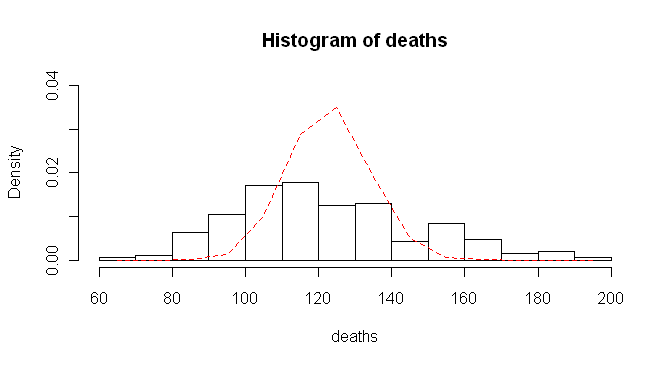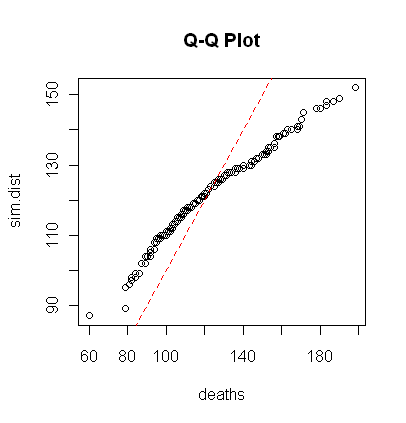| Table of Contents | Function Reference | Function Finder | R Project | COUNTS AND PROPORTIONS Binomial and Poisson Data Count data--data derived from counting things--are often treated as if they are assumed to be binomial distributed or Poisson distributed. The binomial model applies when the counts are derived from independent Bernoulli trials in which the probability of a "success" is known, and the number of trials (i.e., the maximum possible value of the count) is also known. The classic example is coin tossing. We toss a coin 100 times and count the number of times the coin lands heads side up. The maximum possible count is 100, and the probability of adding one to the count (a "success") on each trial is known to be 0.5 (assuming the coin is fair). Trials are independent; i.e., previous outcomes do not influence the probability of success on current or future trials. Another requirement is that the probability of success remain constant over trials. Poisson counts are often assumed to occur when the maximum possible count is not known, but is assumed to be large, and the probability of adding one to the count at each moment (trials are often ill defined) is also unknown, but is assumed to be small. A example may help. Suppose we are counting traffic fatalities during a given month. The maximum possible count is quite high, we can imagine, but in advance we can't say exactly (or even approximately) what it might be. Furthermore, the probability of a fatal accident at any given moment in time is unknown but small. This sounds like it might be a Poisson distributed variable. But is it? The built in data set "Seatbelts" allows us to have a look at such a count variable. "Seatbelts" is not a data frame, it's a time series, so extracting the counts of fatalities will take a bit of trickery. ```> Seatbelts some output not shown DriversKilled drivers front rear kms PetrolPrice VanKilled law Jan 1969 107 1687 867 269 9059 0.10297181 12 0 Feb 1969 97 1508 825 265 7685 0.10236300 6 0 Mar 1969 102 1507 806 319 9963 0.10206249 12 0 Apr 1969 87 1385 814 407 10955 0.10087330 8 0 ... > deaths = as.vector(Seatbelts[,1]) # all rows of column 1 extracted as a vector > length(deaths)  192 > mean(deaths)  122.8021 > var(deaths)  644.1386``` The vector "deaths" now contains the monthly number of traffic deaths in Great Britain during the 192 months from January 1969 through December 1984. We also have our first indication this is not a Poisson distributed variable. In the Poisson distribution, the mean and the variance have the same value. Let's continue nevertheless. Next we'll look at a histogram of the "deaths" vector, and plotted over top of that we will put the Poisson distribution with mean = 122.8. ```> hist(deaths, breaks=15, prob=T, ylim=c(0,.04)) > lines(x<-seq(65,195,10),dpois(x,lambda=mean(deaths)),lty=2,col="red")``` And I'm not gonna lie to you. That took some trial and error! The histogram function asks for 15 break points, turns on density plotting, and sets the y-axis to go from 0 to .04. The poisson density function was plotted using the lines() function. The x-values were generated by using seq() and stored into "x" on the fly. The y-values were generated using the dpois() function. We also requested a dashed, red line. Examination of the figure shows what we suspected. The empirical distribution does not match the theoretical Poisson distribution.```> sim.dist = rpois(192, lambda=mean(deaths)) > qqplot(deaths, sim.dist, main="Q-Q Plot") > abline(a=0, b=1, lty=2, col="red")``` Finally, you may recall (if you read that tutorial) that the qqnorm() function can be used to check a distribution for normality. The qqplot() function will compare any two distributions to see if they have the same shape. If they do, the plotted points will fall along a straight line. The plot to the right doesn't look too terribly bad until we realize these two distributions should not only both be poisson, they should also both have the same mean (or lambda value). Thus, the points should fall along a line with intercept 0 and slope 1. The moral of the story: just because count data sound like they might fit a certain distribution doesn't mean they will. R provides a number of mechanisms for checking this. We could have saved ourselves a lot of trouble by looking at a time series plot to begin with. I will not reproduce it, but the command for doing so is below. In the plot, we see the data are strongly cyclical and, therefore, that the individual elements of the vector should not be considered independent counts. In fact, there is a yearly cycle in the number of traffic deaths. `> plot(Seatbelts[,1]) # Not shown.` Furthermore, a scatterplot of "deaths" against its index values appears to show that the probability of dying in a traffic accident is decreasing over the duration of the record. `> scatter.smooth(1:192, deaths) # Not shown.` So there are lot's of problems with the poisson model here! The Binomial Test Suppose we set up a classic card-guessing test for ESP using a 25-card deck of Zener cards, which consists of 5 cards each of 5 different symbols. If the null hypothesis is correct (H0: no ESP) and the subject is just guessing at random, then we should expect pN correct guesses from N independent Bernoulli trials on which the probability of a success (correct guess) is p = 0.2. Suppose our subject gets 9 correct guesses. Is this out of line with what we should expect just by random chance? A number of proportion tests could be applied here, but the sample size is fairly small (just 25 guesses), so an exact binomial test is our best choice. ```> binom.test(x=9, n=25, p=.2) Exact binomial test data: 9 and 25 number of successes = 9, number of trials = 25, p-value = 0.07416 alternative hypothesis: true probability of success is not equal to 0.2 95 percent confidence interval: 0.1797168 0.5747937 sample estimates: probability of success 0.36``` Oh, too bad! Assuming we set alpha at the traditional value of .05, we fail to reject the null hypothesis with an obtained p-value of .074. The 95% confidence interval tells us this subject's true rate of correct guessing is best approximated as being between 0.18 and 0.57. This incorporates the null value of 0.2, so once again, we must regard the results as being consistent with the null hypothesis. We might argue at this point that we should have done a one-tailed test. (A two-tailed test is the default.) Of course, this decision should be made in advance, but if the subject is displaying evidence of ESP, we would expect his success rate to be not just different from chance but greater than chance. To take this into account in the test, we need to set the "alternative=" option. Choices are "less" than expected, "greater" than expected, and "two.sided" (the default). ```> binom.test(x=9, n=25, p=.2, alternative="greater") Exact binomial test data: 9 and 25 number of successes = 9, number of trials = 25, p-value = 0.04677 alternative hypothesis: true probability of success is greater than 0.2 95 percent confidence interval: 0.2023778 1.0000000 sample estimates: probability of success 0.36``` The one-tailed test allows the null to be rejected at alpha=.05. The confidence interval says the subject is guessing with a success rate of at least 0.202. The confidence level can also be set by changing the "conf.level=" option to any reasonable value less than 1. The default value is .95. The Single-Sample Proportion Test The subject keeps guessing because, of course, we'd like to see this above chance performance repeated. He has now made 400 passes through the deck for a total of 10,000 independent guesses. He has guessed correctly 2,022 times. What should we conclude? An exact binomial test is probably not the best choice here as the sample size is now very large. We'll substitute a single-sample proportion test. ```> prop.test(x=2022, n=10000, p=.2, alternative="greater") 1-sample proportions test with continuity correction data: 2022 out of 10000, null probability 0.2 X-squared = 0.2889, df = 1, p-value = 0.2955 alternative hypothesis: true p is greater than 0.2 95 percent confidence interval: 0.1956252 1.0000000 sample estimates: p 0.2022``` The syntax is exactly the same. Notice the proportion test calculates a chi-squared statistic. The traditional z-test of a proportion is not implemented in R, but the two tests are exactly equivalent. Notice also a correction for continuity is applied. If you don't want it, set the "correct=" option to FALSE. The default value is TRUE. (This option must be set to FALSE to make the test mathematically equivalent to the uncorrected z-test of a proportion.) Two-Sample Proportions Test A random sample of 428 adults from Myrtle Beach reveals 128 smokers. A random sample of 682 adults from San Francisco reveals 170 smokers. Is the proportion of adult smokers in Myrtle Beach different from that in San Francisco? ```> prop.test(x=c(128,170), n=c(428,682), alternative="two.sided", conf.level=.99) 2-sample test for equality of proportions with continuity correction data: c(128, 170) out of c(428, 682) X-squared = 3.0718, df = 1, p-value = 0.07966 alternative hypothesis: two.sided 99 percent confidence interval: -0.02330793 0.12290505 sample estimates: prop 1 prop 2 0.2990654 0.2492669``` The two-proportions test also does a chi-square test with continuity correction, which is mathematically equivalent to the traditional z-test with correction. Enter "hits" or successes into the first vector ("x"), the sample sizes into the second vector ("n"), and set options as you like. To turn off the continuity correction, set "correct=F". I set the alternative to two-sided, but this was unnecessary as two-sided is the default. I also set the confidence level for the confidence interval to 99% to illustrate this option. I made up these data, by the way. R incorporates a function for calculating the power of a 2-proportions test. The syntax is illustrated here from the help page. ```power.prop.test(n = NULL, p1 = NULL, p2 = NULL, sig.level = 0.05, power = NULL, alternative = c("two.sided", "one.sided"), strict = FALSE)``` The value of n should be set to the sample size per group, p1 and p2 to the group probabilities or proportions of successes, and power to the desired power. One and only one of these options must be passed as NULL, and R will calculate it from the others. In the example above, what sample sizes should we have if we want a power of 90%? ```> power.prop.test(p1=.299, p2=.249, sig.level=.05, power=.9, + alternative="two.sided") Two-sample comparison of proportions power calculation n = 1670.065 p1 = 0.299 p2 = 0.249 sig.level = 0.05 power = 0.9 alternative = two.sided NOTE: n is number in *each* group``` We would need 1,670 subjects in each group. Multiple Proportions Test The two-proportions test generalizes directly to a multiple proportions test. The example from the help page should suffice to illustrate. ```> example(prop.test) > ## Data from Fleiss (1981), p. 139. > ## H0: The null hypothesis is that the four populations from which > ## the patients were drawn have the same true proportion of smokers. > ## A: The alternative is that this proportion is different in at > ## least one of the populations. > > smokers <- c( 83, 90, 129, 70 ) > patients <- c( 86, 93, 136, 82 ) > prop.test(smokers, patients) 4-sample test for equality of proportions without continuity correction data: smokers out of patients X-squared = 12.6004, df = 3, p-value = 0.005585 alternative hypothesis: two.sided sample estimates: prop 1 prop 2 prop 3 prop 4 0.9651163 0.9677419 0.9485294 0.8536585``` If you run this example, you will notice that I have deleted some of the output from the simpler proportion tests. revised 2016 January 27 | Table of Contents | Function Reference | Function Finder | R Project |